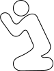CodePlea
Random thoughts on programming
30 Nov 2016

# Interpolating in a Triangle

Let's say we have a triangle. Maybe something like this:

Furthermore, let's say that each vertex (corner) has an associated value of some sort. For example: assume each vertex has an associated color. Perhaps we have:

• Vertex 1 is red
• Vertex 2 is green
• Vertex 3 is purple

Given an arbitrary point, P, inside of the triangle, how do we define its color?

We're using color as only an example. We may actually want to interpolate over any attribute associated at the vertexes. e.g. U/V texture coordinates, Z-buffer depth, normal values.

If you played the video game SimAnt before, you may remember the behavior control using a triangle to balance a ratio of three actions: forage, dig, and nurse. This is another example of interpolating over the triangle.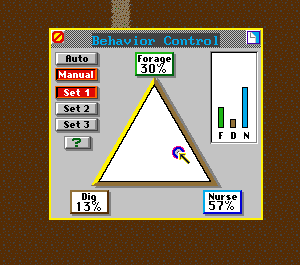## The Lazy Approach

We could simply say that P is closest to V1, and therefore make P red. If we do this for every point in the triangle, we get this: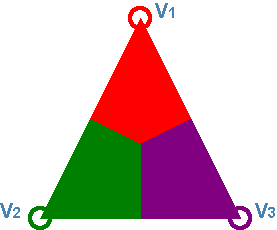This is called the Nearest-Neighbor method, and it gives us something call a Voronoi diagram. In any case, it's not what we're looking for.

## The Naive Solution

I'd like to work through one other false start before giving the final answer. I find that when the solution is a bit complicated, it's nice to first justify why a simpler approach won't work.

So a point in the triangle should take some value from all three of the vertices. We know that it should take more value from vertices that it's closer to, and less value from ones that it's farther away from. In our earlier example, we can see that our query point, P, is fairly close to V1 and V2. It's a good deal farther from V3. So it makes sense to think that we should color it more red and green and less purple.

We can figure the distance from P to each vertex by using the Pythagorean theorem.

$$Distance_{v1} = \sqrt{(X_{v1} - P_{x})^2 + (Y_{v1} - P_{y})^2}$$
$$Distance_{v2} = \sqrt{(X_{v2} - P_{x})^2 + (Y_{v2} - P_{y})^2}$$
$$Distance_{v3} = \sqrt{(X_{v3} - P_{x})^2 + (Y_{v3} - P_{y})^2}$$

Distances give us big numbers when we are far away, and small numbers when we're close. So we might try inverting them so that bigger numbers give more weight to closer verticies. In other words, we'll interpolate inversely proportional to the distance to each vertex.

So we could define our weights as:

$$W_{v1} = \frac{1}{Distance_{v1}}$$
$$W_{v2} = \frac{1}{Distance_{v2}}$$
$$W_{v3} = \frac{1}{Distance_{v3}}$$

Now we can define our color as a weighted average:

$$Color_{p} = \frac{W_{v1} Color_{v1} + W_{v2} Color_{v2} + W_{v3} Color_{v3}} {W_{v1} + W_{v2} + W_{v3}}$$

This has the nice property that a vertex twice as far away only contributes half as much to the solution. This techinque is useful in many other fields too. For example, in machine learing it is common to normalize a distance metric by taking its inverse.

So in summary, we are finding an interpolated color at point P by blending the vertex colors in porportion to how close they are to P.

You can mouse around in this demo to see how the weight values change inside the triangle with this method.

Using this method for color interpolation over the entire triangle yields: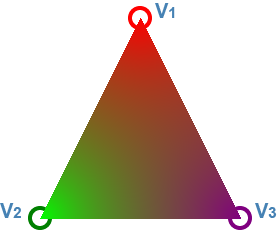Doesn't look too bad, eh? This solution is simple, easy to implement, and fairly intuitive. It would probably work fine in many applications. However, if we shift the triangle a bit, we see the major problem with this approach.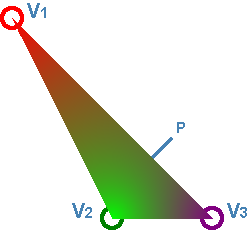Look at point P in the example above. It lies directly on the straight line between V1 and V3. For most interpolation problems, we would actually like the value at P to be a combination solely of V1 and V3. We would usually ignore V2 completely at point P. With our naive solution, however, we've really messed up! Since point P is closest to V2, P ends up taking most of its value from V2!

If this doesn't seem like a big deal, just think of how you would use interpolated data on a triangle. For example, if you were interpolating a Z-buffer coordinate, you would expect any point on the line between V1 and V3 to use only the Z coordinates of V1 and V3. If it used any of V2, then it wouldn't be a straight line!

Take another look at SimAnt. If we set the cursor exactly between forage and dig, we see that the nurse weight is 0. So SimAnt has a better method than our naive inverse weighted distance method.Let's try another approach.

## Barycentric Coordinates

The real solution to our problem is Barycentric Coordinates. The concept can be a bit subtle to grasp at first, but it's not complicated, and it will make sense with just a bit of pondering.

The trick to Barycentric Coordinates is to find the weights for V1, V2, and V3 that balance the following system of equations:

$$P_{x} = W_{v1} X_{v1} + W_{v2} X_{v2} + W_{v3} X_{v3}$$
$$P_{y} = W_{v1} Y_{v1} + W_{v2} Y_{v2} + W_{v3} Y_{v3}$$
$$W_{v1} + W_{v2} + W_{v3} = 1$$

Think about what those equations mean for a minute. What we're really asking for is self-consistancy. If we want to interpolate color or Z-buffer values, we're asking for weights that would also work for the X and Y coordinates themselves in the same consistant manner! In other words, for a given point P, we're finding weights that tell us how much of P's X coordinate is made of V1, V2, and V3, and also the same for P's Y coordinate.

With a lot of rearranging, we can solve for W1, W2, and W3 for any given point with the following equations:

$$W_{v1}=\frac{(Y_{v2}-Y_{v3})(P_{x}-X_{v3})+(X_{v3}-X_{v2})(P_{y}-Y_{v3})}{(Y_{v2}-Y_{v3})(X_{v1}-X_{v3})+(X_{v3}-X_{v2})(Y_{v1}-Y_{v3})}$$
$$W_{v2}=\frac{(Y_{v3}-Y_{v1})(P_{x}-X_{v3})+(X_{v1}-X_{v3})(P_{y}-Y_{v3})}{(Y_{v2}-Y_{v3})(X_{v1}-X_{v3})+(X_{v3}-X_{v2})(Y_{v1}-Y_{v3})}$$
$$W_{v3}=1 - W_{v1} - W_{v2}$$

Note that the denominators are the same for W1 and W2, and also that W3 is calculated by the fact that all of the weights sum to 1.

You can mouse around in this demo to see how the weight values change inside the triangle.

Another thing about barycentric coordinates, is that if P is actually outside of the triangle, then at least one of W1, W2, or W3 will be negative! This can be used as an easy check to see if a point lies inside or outside of a triangle.

In fact, a common triangle drawing algorithm is to look at every pixel in a bounding box around the triangle. Then for each pixel, calculate the barycentric coordinates (which are needed anyway to interpolate the depth buffer, texture coordinates, etc). If one of the weights is a negative number, then the pixel is simply skipped. One advantage of this algorithm is that a graphics card can simply parallelize every pixel in the bounding box. This allows drawing triangles to be very fast.

Here's what the final color interpolation from our original question looks like if we use a barycentric coordinate system.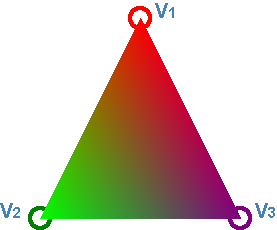I hope you found that build-up and explanation to barycentric coordinates interesting. Let me know what you think by leaving a comment below.

Like this post? Consider following me on Twitter or following me on Github. Don't forget to subscribe to my feed.